## RS Aggarwal Class 8 Solutions Chapter 1 Rational Numbers Ex 1F

These Solutions are part of RS Aggarwal Solutions Class 8. Here we have given RS Aggarwal Solutions Class 8 Chapter 1 Rational Numbers Ex 1F.

Other Exercises

Question 1.
Solution:
Rational number between $$\\ \frac { 1 }{ 4 }$$ and $$\\ \frac { 1 }{ 3 }$$
= $$\\ \frac { 1 }{ 2 }$$ $$\left[ \frac { 1 }{ 4 } +\frac { 1 }{ 3 } \right]$$
= $$\\ \frac { 1 }{ 2 }$$ $$\left[ \frac { 3+4 }{ 12 } \right]$$
= $$\\ \frac { 1 }{ 2 }$$ x $$\\ \frac { 7 }{ 12 }$$
= $$\\ \frac { 7 }{ 24 }$$

Question 2.
Solution:
Solution. Rational number between 2 and 3
= $$\\ \frac { 1 }{ 2 }$$(2 + 3)
= $$\\ \frac { 1 }{ 2 }$$ x 5
= $$\\ \frac { 5 }{ 2 }$$

Question 3.
Solution:
Rational number between $$\\ \frac { -1 }{ 3 }$$ and $$\\ \frac { 1 }{ 2 }$$
= $$\\ \frac { 1 }{ 2 }$$ $$\left[ \frac { -1 }{ 3 } +\frac { 1 }{ 2 } \right]$$
= $$\\ \frac { 1 }{ 2 }$$ $$\left[ \frac { -2+3 }{ 6 } \right]$$
= $$\\ \frac { 1 }{ 2 }$$ x $$\\ \frac { 1 }{ 6 }$$
= $$\\ \frac { 1 }{ 12 }$$

Question 4.
Solution:
First rational number between -3 and -2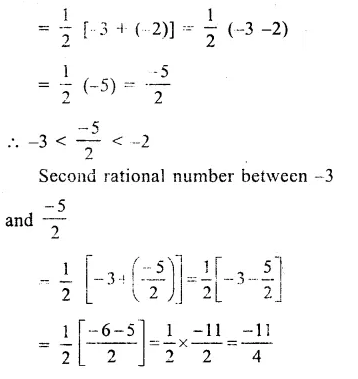Question 5.
Solution:
First rational number between 4 and 5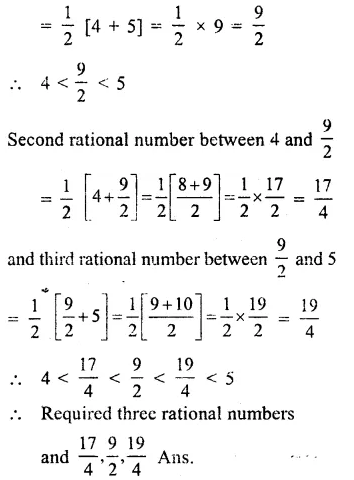Question 6.
Solution:
First rational numbers between $$\\ \frac { 2 }{ 3 }$$ and $$\\ \frac { 3 }{ 4 }$$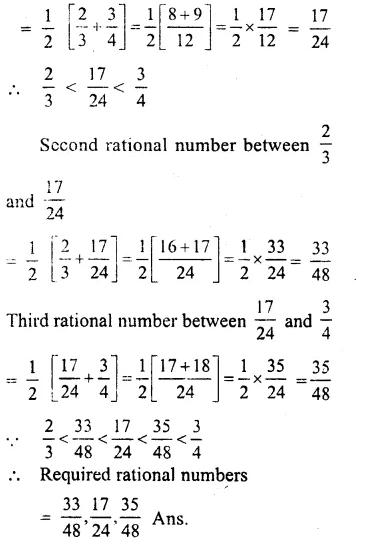Question 7.
Solution: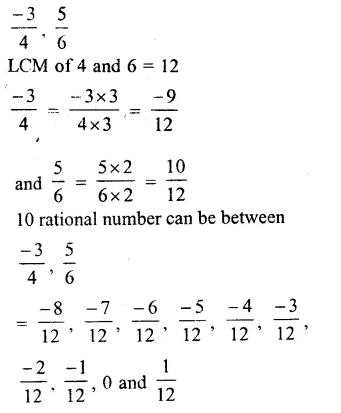Question 8.
Solution:
– 1 = $$\\ \frac { -5 }{ 5 }$$ and 2 = $$\\ \frac { 10 }{ 5 }$$
12 rational number between – 1 and 2 can be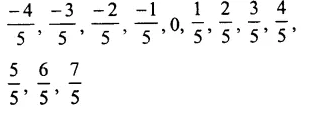Hope given RS Aggarwal Solutions Class 8 Chapter 1 Rational Numbers Ex 1F are helpful to complete your math homework.

If you have any doubts, please comment below. Learn Insta try to provide online math tutoring for you.